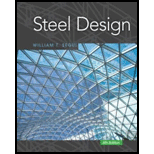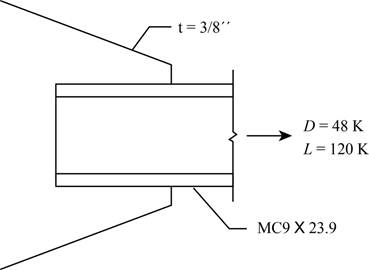# Design a welded connection for an MC 9 × 23.9 of A572 Grade 50 steel connected to a 3 8 -inch-thick gusset plate. The gusset plate is A36 steel. Show your results on a sketch, complete with dimensions. a . Use LRFD. b . Use ASD.### Steel Design (Activate Learning wi...

6th Edition
Segui + 1 other
Publisher: Cengage Learning
ISBN: 9781337094740

#### Solutions

Chapter
Section### Steel Design (Activate Learning wi...

6th Edition
Segui + 1 other
Publisher: Cengage Learning
ISBN: 9781337094740
Chapter 7, Problem 7.11.7P
Textbook Problem
7 views

## Design a welded connection for an MC 9 × 23.9 of A572 Grade 50 steel connected to a 3 8 -inch-thick gusset plate. The gusset plate is A36 steel. Show your results on a sketch, complete with dimensions.a. Use LRFD.b. Use ASD.To determine

(a)

The design of a welded connection using Load and Resistance Factor Design (LRFD).

### Explanation of Solution

Given:

Figure-(1)

Concept Used:

Write the expression for the maximum size of the weld.

wmax=t116in     ...... (I)

Here, the maximum size of the weld is wmax, the thickness of the member is t.

Write the expression for the minimum size of the weld.

wmin=14in

Write the expression for the required length of the weld in longitudinal direction.

Ll=LreqLt2     ...... (II)

Here, the length of the weld in the longitudinal direction is Ll, the required length of the weld is Lreq, the length of the weld in the transverse direction is Lt.

Write the expression for the required length of the weld.

Lreq=PuϕRn     ...... (III)

Here, the factored load is Pu, the load reduction factor is ϕ, the design strength of the connection is Rn.

Write the expression for the factored load.

Pu=1.2D+1.6L     ...... (IV)

Write the nominal weld strength equation per unit length.

wn=ϕRnn     ...... (V)

Here, the nominal weld strength is wn, the nominal strength of the weld per unit length is Rnn.

Write the expression for the nominal strength of the weld per unit length.

Rnn=0.707w(0.6FEXX)     ...... (VI)

Here, the weld size is w, the strength of the weld material is FEXX.

Write the expression for the shear strength of the base metal in yielding.

Puy=1.0(0.6Fyt)     ...... (VII)

Here, the shear strength of the base metal in yielding is Puy, the strength of the weld in yielding is Fy,

Write the expression for the shear strength of the base metal in rupture.

Pur=0.75(0.6Fut)     ...... (VIII)

Here, the shear strength of the base metal in rupture is Pur, the strength of the weld in rupture is Fu.

Write the expression for the longitudinal length of the weld.

Ll=Pl2wl     ...... (IX)

Here, the capacity of weld in longitudinal direction is Pl and the size of the weld in longitudinal direction is wl.

Write the expression for the capacity of the longitudinal weld.

Pl=PuLtwt     ...... (X)

Here, the load capacity of the weld is wt.

Write the expression for the load capacity of the weld in longitudinal direction.

wl=0.85ϕRn     ....... (XI)

Here, the load capacity of the weld in longitudinal direction.

Write the expression for the load capacity of the weld in transverse direction.

wt=1.5ϕRn     ...... (XII)

Here, the load capacity of the weld in transverse direction.

Check the strength of the weld in block shear.

Write the expression for the block shear.

Rreq=0.6FyAnv+UbsFuAnt     ...... (XIII)

Here, the required strength of the weld is Rreq, the gross area along the longitudinal direction is Agv, the net area along the transverse direction is Ant, the load reduction factor is Ubs.

Write the expression for the gross area of the weld.

Agv=Anv=2tLl     ...... (XIV)

Write the expression for the net area of the weld.

Ant=tLt     ...... (XV)

Calculation:

Calculate the maximum size of the weld.

Substitute 38in for t in the Equation (I).

wmax=38in116in=516in

Calculate the minimum size of the weld.

wmin=316in

Hence, adopt weld of thickness 316in of E70XX material.

Substitute 48kips for D and 120kips for L in the Equation (IV).

Pu=(1.2×48kips)+(1.6×120kips)=249.6kips

Calculate the nominal strength of the weld per unit length.

Substitute 14in for w, 70ksi for FEXX in the Equation (VI).

Rnn=0.707×14×(0.6×70ksi)=7.424kips/in

Calculate the nominal weld strength equation per unit length.

Substitute 0.75 for ϕ, 7.424kips/in for Rnn in the Equation (V).

wn=0.75×7.424kips/in=5.568kips/in

Calculate the shear strength of the base metal in yielding.

Substitute 36ksi for Fy, 38in for t in the Equation (VII).

Puy=1.0×(0.6×36ksi×38in)=8.1kips/in

Calculate the shear strength of the base metal in rupture.

Substitute 50ksi for Fy, 38in for t in the Equation (VIII).

Pur=0.75×(0.6×50ksi×38in)=8.438kips/in

The strength of the base metal is the minimum of the shear strength in yielding and rupture.

Thus, the strength of the base metal is 8

To determine

(b)

The design of a welded connection using Allowable Strength Design (ASD).

### Still sussing out bartleby?

Check out a sample textbook solution.

See a sample solution

#### The Solution to Your Study Problems

Bartleby provides explanations to thousands of textbook problems written by our experts, many with advanced degrees!

Get Started

Find more solutions based on key concepts
Determine the mass moment of inertia for the following objects: a thin disk, circular cylinder, and a sphere. R...

Engineering Fundamentals: An Introduction to Engineering (MindTap Course List)

What is the purpose of a CHECK constraint?

Database Systems: Design, Implementation, & Management

What is source data automation? What benefits can it be expected to deliver?

Principles of Information Systems (MindTap Course List)

Why do companies hold short-term investments?

Cornerstones of Financial Accounting

What are referential constraint actions?

Database Systems: Design, Implementation, & Management

Describe two safety precautions you should observe when using layout dye.

Precision Machining Technology (MindTap Course List)

Linux is proprietary software.

Enhanced Discovering Computers 2017 (Shelly Cashman Series) (MindTap Course List)

What is a GFCI?

Welding: Principles and Applications (MindTap Course List)

If your motherboard supports ECC DDR3 memory, can you substitute non-ECC DDR3 memory?

A+ Guide to Hardware (Standalone Book) (MindTap Course List)